Mayur Badole — May 6, 2021
This article was published as a part of the Data Science Blogathon.

In this article, we will learn about how can we able to handle multi categorical variables using the Feature Engineering technique One Hot Encoding.

But before going ahead, let us have a brief discussion on Feature engineering and One Hot Encoding.

## Feature Engineering

So, Feature Engineering is the process of extracting features from raw data using the domain knowledge of the problem. These features can be used to improve the performance of machine learning algorithms and if the performance increase then it will give the best accuracy. We can also say that feature engineering is the same as applied machine learning. Feature engineering is the most important art in machine learning which creates a huge difference between a good model and a bad model. This is the third step in any data science project life cycle.

The concept of transparency for the machine learning models is a complicated thing as different models often require different approaches for the different kinds of data. Such as:-

• Continuous data
• Categorical features
• Missing values
• Normalization
• Dates and time

But here we will only discuss Categorical Features, The Categorical Features are those features in which datatype is an Object type. The value of data point in any categorical feature is not in numerical form, rather it was in object form.

There are many techniques for handling the categorical variables, some are :

• Label Encoding or Ordinal Encoding
• One hot Encoding
• Dummy Encoding
• Effect Encoding
• Binary Encoding
• Basel Encoding
• Hash Encoding
• Target Encoding

So, here we handling categorical features by One Hot Encoding, thus first of all we will discuss One Hot Encoding.

## One Hot Encoding

We know that the categorical variables contain the label values rather than numerical values. The number of possible values is often limited to a fixed set. Categorical variables are often called nominal. Many machine learning algorithms cannot operate on label data directly. They require all input variables and output variables to be numeric.

This means that categorical data must be converted to a numerical form. If the categorical variable is an output variable, you may also want to convert predictions by the model back into a categorical form to present them or use them in some application.

for example data on gender is in form of ‘male’ and ‘female’.

But if we use one-hot encoding then encoding and allowing the model to assume a natural ordering between categories may result in poor performance or unexpected results.

One-hot encoding can be applied to the integer representation. This is where the integer encoded variable is removed and a new binary variable is added for each unique integer value.

For example, we encode colors variable,

 Red_color Blue_color 0 1 1 0 0 1

Now we will start our journey. In the first step, we take a dataset of house price prediction.

## Dataset

Here we will use the dataset of house_price which is used in predicting the house price according to the size of the area.

## Importing Modules

Now, we have to import important modules from python that will use for the one-hot encoding

```# importing pandas
import pandas as pd
# importing numpy
import numpy as np
# importing OneHotEncoder
from sklearn.preprocessing import OneHotEncoder()```

Here, we use pandas which are used for data analysis, NumPyused for n-dimensional arrays, and from sklearn, we will use one important class One Hot Encoder for categorical encoding.

Now we have to read this data using Python.

Generally, the dataset is in the form of CSV, and the dataset we use is also in the form of CSV. For reading CSV file we will use pandas read_csv() function. see below:

```# reading dataset

output:-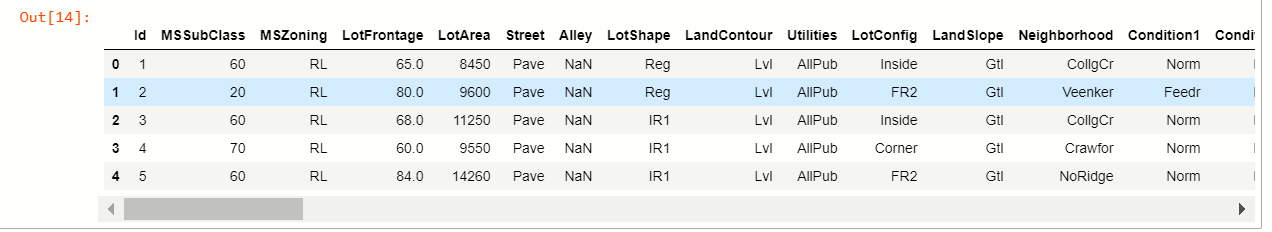But we have to only use categorical variables for one hot encoder and we will only try to explain with categorical variables for easy understanding.

for partitioning categorical variables from data we have to check how many features have categorical values.

## Checking Categorical Values

For checking values we use the pandas select_dtypes function which is used for selecting the data types of variable.

```# checking features
cat = df.select_dtypes(include='O').keys()
# display variabels
cat```

output:-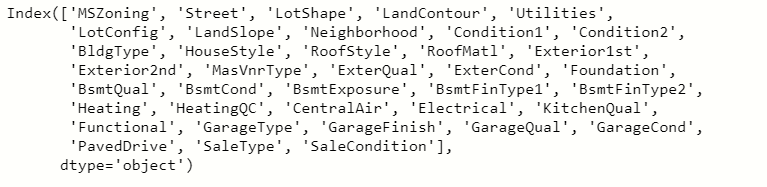Now we have to drop those numerical columns from the dataset and we will use this categorical variable for our use. We only use 3-4 categorical columns from the dataset for applying one-hot encoding.

## Creating New DataFrame

Now, for using categorical variables we will create a new dataframe of selected categorical columns.

```# creating new df
# setting columns we use

output:-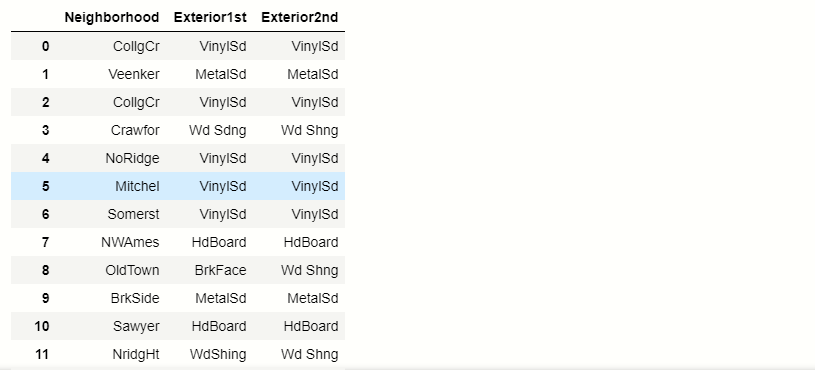Now we have to find out how many unique categories are present in every categorical column.

## Finding Unique Values

For finding unique values we will use pandas unique() function.

```# unique values in each columns
for x in new_df.columns:
#prinfting unique values
print(x ,':', len(new_df[x].unique()))```

output:-

 Neighborhood : 25 Exterior1st : 15 Exterior2nd : 16

Now, we will go for our technique to apply one-hot encoding on multi categorical variables.

## Technique For Multi Categorical Variables

The technique is that we will limit one-hot encoding to the 10 most frequent labels of the variable. This means that we would make one binary variable for each of the 10 most frequent labels only, this is equivalent to grouping all other labels under a new category, which in this case will be dropped. Thus, the 10 new dummy variables indicate if one of the 10 most frequent labels is present is 1 or not then 0 for a particular observation.

### Most Frequent variables

Here we will select the 20 most frequent variables.

Suppose we take one categorical variable Neighborhood.

```# finding the top 20 categories

output: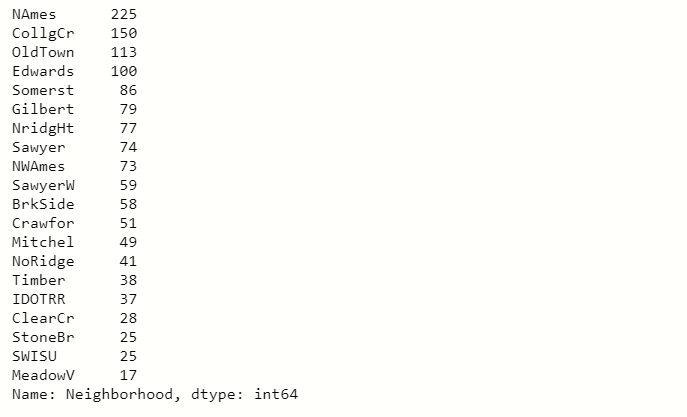When you see in this output image you will notice that the NAmes label is repeating 225 times in the Neighborhood columns and we go down this number is decreasing.

So we took the top 10 results from the top and we convert this top 10 result into one-hot encoding and the left labels turn into zero.

output:-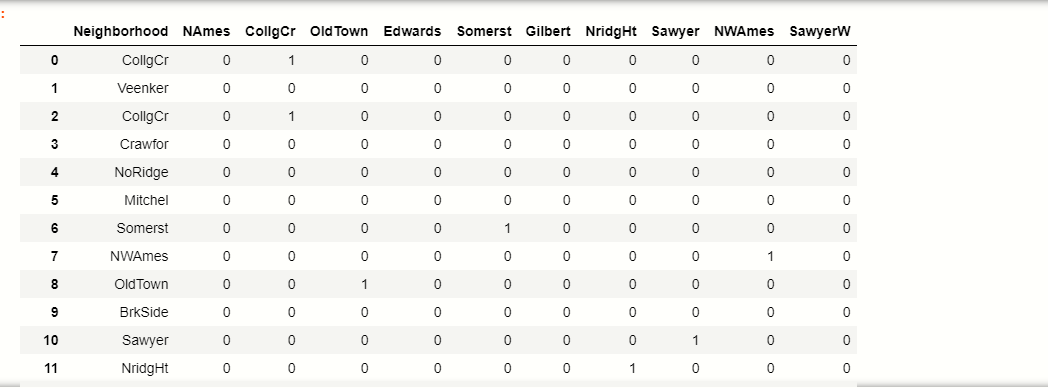### List of Most Frequent Categorical Variables

```# make list with top 10 variables
top_10 = [x for x in new_df.Neighborhood.value_counts().sort_values(ascending=False).head(10).index]
top_10```

output:-

[‘NAmes’,
‘CollgCr’,
‘OldTown’,
‘Edwards’,
‘Somerst’,
‘Gilbert’,
‘NridgHt’,
‘Sawyer’,
‘NWAmes’,
‘SawyerW’]

There are the top 10 categorical labels in the Neighborhood column.

### Make Binary

Now, we have to make the 10 binary variables of the top_10 labels:

# make binary of labels
for label in top_10:
new_df[label] = np.where(new_df[‘Neighborhood’]==label,1,0)
new_df[[‘Neighborhood’]+top_10]

output:-

NAmes CollgCr OldTown Edwards Somerst Gilbert NridgHt Sawyer NWAmes SawyerW
0 CollgCr 0 1 0 0 0 0 0 0 0 0
1 Veenker 0 0 0 0 0 0 0 0 0 0
2 CollgCr 0 1 0 0 0 0 0 0 0 0
3 Crawfor 0 0 0 0 0 0 0 0 0 0
4 NoRidge 0 0 0 0 0 0 0 0 0 0
5 Mitchel 0 0 0 0 0 0 0 0 0 0
6 Somerst 0 0 0 0 1 0 0 0 0 0
7 NWAmes 0 0 0 0 0 0 0 0 1 0
8 OldTown 0 0 1 0 0 0 0 0 0 0
9 BrkSide 0 0 0 0 0 0 0 0 0 0
10 Sawyer 0 0 0 0 0 0 0 1 0 0
11 NridgHt 0 0 0 0 0 0 1 0 0 0

You can see how the top_10 labels are now converted into binary format.

Let take an example, see in the table where 1 index Veenker which was not belonging to our top_10 categories label so it will result in 0 all the columns.

Now we will do it for all the Categorical variables that we have selected above.

### All Selected variable on OneHotEncoding

```# for all categorical variables we selected
def top_x(df2,variable,top_x_labels):
for label in top_x_labels:
df2[variable+'_'+label] = np.where(data[variable]==label,1,0)
#encode Nighborhood into the 10 most frequent categories
top_x(data,'Neighborhood',top_10)
# display data

Output:-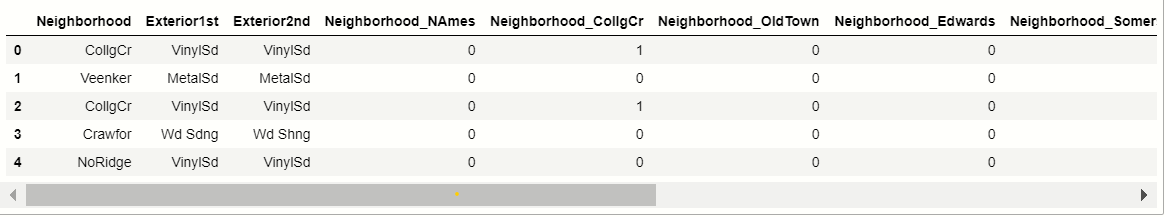Now, here we apply the one-hot encoding on all multi categorical variables.

Now we will see the advantages and disadvantages of One Hot Encoding for multi variables.

• Straightforward to implement
• Does not require much time for variable exploration
• Does not expand massively the feature space.

• Does not add any information that may make the variable more predictive
• Do not keep the information of the ignored variables.

## End Notes

So, the Summary of this is that we learn about how to handle multi categorical variables, If you come across this problem then this is a very difficult task. So thank you for reading this article.

Connect with me on Linkedin: Profile